Introduction to Statistical Modeling with SAS/STAT Software

### Bayesian Models

Statistical models based on the classical (or frequentist) paradigm treat the parameters of the model as fixed, unknown constants. They are not random variables, and the notion of probability is derived in an objective sense as a limiting relative frequency. The Bayesian paradigm takes a different approach. Model parameters are random variables, and the probability of an event is defined in a subjective sense as the degree to which you believe that the event is true. This fundamental difference in philosophy leads to profound differences in the statistical content of estimation and inference. In the frequentist framework, you use the data to best estimate the unknown value of a parameter; you are trying to pinpoint a value in the parameter space as well as possible. In the Bayesian framework, you use the data to update your beliefs about the behavior of the parameter to assess its distributional properties as well as possible.

Suppose you are interested in estimating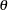from data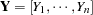by using a statistical model described by a density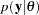. Bayesian philosophy states thatcannot be determined exactly, and uncertainty about the parameter is expressed through probability statements and distributions. You can say, for example, thatfollows a normal distribution with meanand variance, if you believe that this distribution best describes the uncertainty associated with the parameter.

The following steps describe the essential elements of Bayesian inference:

1. A probability distribution foris formulated as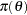, which is known as the prior distribution, or just the prior. The prior distribution expresses your beliefs, for example, on the mean, the spread, the skewness, and so forth, about the parameter prior to examining the data.

2. Given the observed data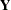, you choose a statistical modelto describe the distribution ofgiven.

3. You update your beliefs aboutby combining information from the prior distribution and the data through the calculation of the posterior distribution,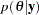.

The third step is carried out by using Bayes’ theorem, from which this branch of statistical philosophy derives its name. The theorem enables you to combine the prior distribution and the model in the following way: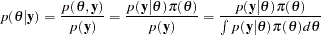The quantity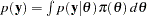is the normalizing constant of the posterior distribution. It is also the marginal distribution of, and it is sometimes called the marginal distribution of the data.

The likelihood function ofis any function proportional to—that is,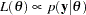. Another way of writing Bayes’ theorem is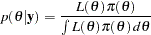The marginal distribution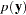is an integral; therefore, provided that it is finite, the particular value of the integral does not yield any additional information about the posterior distribution. Hence,can be written up to an arbitrary constant, presented here in proportional form, as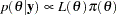Bayes’ theorem instructs you how to update existing knowledge with new information. You start from a prior belief, and, after learning information from data, you change or update the belief onand obtain. These are the essential elements of the Bayesian approach to data analysis.

In theory, Bayesian methods offer a very simple alternative to statistical inference—all inferences follow from the posterior distribution. However, in practice, only the most elementary problems enable you to obtain the posterior distribution analytically. Most Bayesian analyses require sophisticated computations, including the use of simulation methods. You generate samples from the posterior distribution and use these samples to estimate the quantities of interest.

Both Bayesian and classical analysis methods have their advantages and disadvantages. Your choice of method might depend on the goals of your data analysis. If prior information is available, such as in the form of expert opinion or historical knowledge, and you want to incorporate this information into the analysis, then you might consider Bayesian methods. In addition, if you want to communicate your findings in terms of probability notions that can be more easily understood by nonstatisticians, Bayesian methods might be appropriate. The Bayesian paradigm can provide a framework for answering specific scientific questions that a single point estimate cannot sufficiently address. On the other hand, if you are interested in estimating parameters and in formulating inferences based on the properties of the parameter estimators, then there is no need to use Bayesian analysis. When the sample size is large, Bayesian inference often provides results for parametric models that are very similar to the results produced by classical, frequentist methods.